# How to Round Whole Numbers | Rules and Examples

1. Math Lessons >
2. Rounding Whole Numbers

### What Is Rounding?

Rounding is the process of adjusting digits to make calculations easy and quick.

In this lesson, we’ll show you how to round off whole numbers to the nearest 10, to the nearest 100, to the nearest 1,000 and so on, using a simple trick.

### Why Do We Round Numbers?

Let’s think of this scenario:

Debby bought branded accessories worth \$212 and electronic gadgets worth \$789. What is the total of her purchases?

Adding these two amounts: \$212 and \$789, is taking Debby an awful lot of time.

But what if the prices were \$200 and \$800? Debby would be far better off as she could add the amounts in a split second and pay for her purchases with \$1,000.

What we did here was rounding. Rounding makes numbers simpler and easier to understand.

### Rounding Using a Number Line

Let’s take our rounding know-how a notch up by learning how to round using a number line. Let’s understand the underlying place value concept.

We'll try rounding off the number 26 to its nearest 10.

Tens
Ones
2
6

We have 2 in the tens place and 6 in the ones place.

We know that 26 is more than 2 tens but less than 3 tens. Therefore, 20 and 30 become our benchmarks.

Now, we need to decide if we will round down to 20 or round up to 30. Let’s put that on our number line.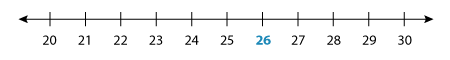We can see that 26 is between 20 and 30. We also see that it’s much closer to 30.

Let’s animate our number line and make it look like a little number hill.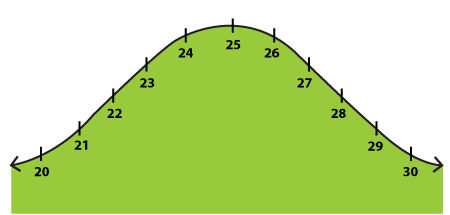Let’s imagine a log is dropped at 26. Which side will it roll to?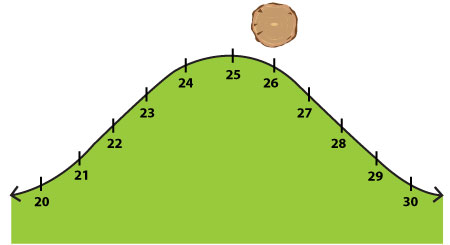Set the rock rolling with a click!

Obviously, to 30! Now that implies we need to round up.

But what if the log was dropped at 22? 22 is closer to 20. Look at the number hill with the log at 22.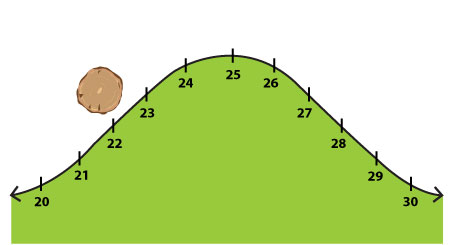Watch the rock roll down with a click!

It is clear that the log would roll to 20. So here we are rounding down.

But is there a way of rounding off without a number line? Yes! There are some simple rules using which we can round off numbers.

Let’s take a look at some examples and learn how rounding up and rounding down work.

### How to Round Numbers to the Nearest 10

Let’s try rounding the number 154 to the nearest 10.

First you need to figure out the digit you are going to round off the number to. This is your target digit.

In this case, we need to identify the digit in the tens place.

Hundreds
Tens
Ones
1
5
4

We have the number 5 in the 10s place here.

With that done, shift your focus to the digit that is on its right.

Here, the digit to the right of 5 is 4.

If you see a 0, 1, 2, 3, or 4 (a number < 5), keep the targeted digit unchanged and drop all the digits on the right of the targeted digit to zero. This is rounding down.

Thus, 154 rounds down to 150.

But, what if you need to round 157 to the nearest 10?

Hundreds
Tens
Ones
1
5
7

Here our target digit is 5, and there’s 7 on its right.

If you see a 5, 6, 7, 8, or 9 (a number ≥ 5), add 1 to the targeted digit and change all digits on the right of the targeted digit to zero. This is rounding up.

So, when we add 1 to the targeted digit, we get:

5 + 1 = 6, which is the new digit in our tens place.

Thus, 157 rounds up to 160.

### How to Round Numbers to the Nearest 100

Now let’s move on to rounding a 4-digit number 3,895 to the nearest 100.

Thousands
Hundreds
Tens
Ones
3
8
9
5

The digit in the 100s place is 8. Let's look at the number to the right of our targeted digit - the 10s place. There is a 9 there, so we need to round up.

Adding 1 to 8, we get 9. And all the digits to the right of 9 are zeros.

Thus, 3,895 rounds up to 3,900.

### How to Round Numbers to the Nearest 1,000

Let’s move one more rung up the ladder and try rounding 29,476 to the closest 1,000.

Ten Thousands
Thousands
Hundreds
Tens
Ones
2
9
4
7
6

The digit in the 1,000s place is 9. The digit to its right is 4.

Should we round down or up? Yes, we should round down.

While rounding down, our targeted digit stays the same and all the digits to its right become zero.

Thus, 29,476 rounds down to 29,000.

Let’s try rounding off 9,507 to the nearest 10, 100 and 1,000.

Thousands
Hundreds
Tens
Ones
9
5
0
7
To the Nearest 10
To the Nearest 100
To the Nearest 1,000

Target place: 10s

Target digit: 0

The digit to its right: 7

7 > 5

∴ Targeted digit + 1 = 0 + 1 = 1.

9,507 rounds up to 9,510.

Target place: 100s

Target digit: 5

The digit to its right: 0

0 < 5

∴ Targeted digit remains the same.

9,507 rounds down to 9,500.

Target place: 1,000s

Target digit: 9

The digit to its right: 5

5 = 5

∴ Targeted digit + 1 = 9 + 1 = 10.

9,507 rounds up to 10,000.

### How to Round Numbers to Any Place

Let’s press on and learn to round multi-digit whole numbers to the nearest 10,000, 100,000, and 1,000,000. All we need to do is follow the usual steps: choose the target digit, check if the digit to its right is < or ≥ 5, and round the number accordingly.

Let’s take a 7-digit number 7,849,625 this time. Here’s its place value table.

Millions
Hundred Thousands
Ten Thousands
Thousands
Hundreds
Tens
Ones
7
8
4
9
6
2
5

Now, let’s whizz through and round the number to each place.

To the Nearest Ten: The digit in the ones place is 5. Rounding the number up, we have 7,849,630.

To the Nearest Hundred: The digit in the tens place is 2. Rounding the number down, we have 7,849,600.

To the Nearest Thousand: The digit in the hundreds place is 6. Rounding up, we have 7,850,000.

To the Nearest Ten Thousand: The digit in the thousands place is 9. Adding 1 to the next digit 4, we have 7,850,000. As you can see, rounding to 1,000 and 10,000 are the same in this case.

To the Nearest Hundred Thousand: The digit in the ten thousands place is 4. So, we need to round the number down this time. We have 7,800,000.

To the Nearest Million: The digit in the hundred thousands place is 8. Thus rounding the number up, we have 8,000,000.

### A Rundown on the Lesson!

• Rounding is adjusting the digits of a number, making the calculations simpler and quicker.

• Follow these rounding rules to round numbers:

• Identify the digit you are going to round off the number to. This is your target digit.

• If the digit to the right of the targeted digit is greater than or equal to 5, round the number up.

• If the digit to its right is less than 5, round the number down.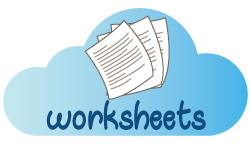Perfect your rounding skills with our free printable Rounding worksheets!

Progress
Score

Restart Quiz